# JEE Main 2017 Physics Paper With Solutions (April 2)

JEE Main 2017 Paper with Solutions Physics - Offline Exam consists of step by step solutions, which enables the students to prepare efficiently for the exams. At BYJU’S, the solutions are prepared accurately and in an easy way by our subject experts. Practising these questions will encourage the students to face the exam confidently. These papers will enhance the time management skills of the students. The solutions uploaded by BYJU’S acts as an effective aid for the students in their preparation and are available in just one click.

### April 2nd - Physics

Question 1: A man grows into a giant such that his linear dimensions increase by a factor of 9. Assuming that his density remains the same, the stress in the leg will change by a factor of :

1. 1) 9
2. 2) 1 / 9
3. 3) 81
4. 4) 1 / 81

Solution:

vf / vi = 93

∵ Density remains same

So, mass ∝ Volume

mf / mi = 93

(Area)f / (Area)i = 92

Stress = (Mass) * g / Area

σ2 / σ1 = (mf / mi) / (Ai / Af)

= 93 / 92

= 9

Question 2: A body is thrown vertically upwards. Which one of the following graphs correctly represents the velocity vs time?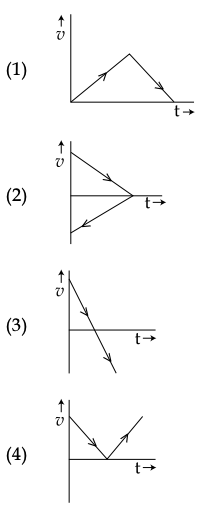Solution:

Acceleration is constant and negative.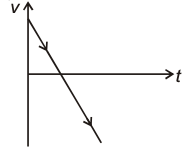Question 3: A body of mass m = 10−2 kg is moving in a medium and experiences a frictional force F = −kv2. Its initial speed is v0 = 10 ms−1. If, after 10 s, its energy is 1 / 8 mv02, the value of k will be:

1. 1) 10−3 kg m−1
2. 2) 10−3 kg s−1
3. 3) 10−4 kg m−1
4. 4) 10−1 kg m−1 s−1

Solution:

kf / ki = (1 / 8) mvo2 / (1 / 2) mvo2 = 1 / 4

vf / vi = 1 / 2

vf = vo / 2

-kv2 = mdv / dt

v0v0/2 dv / v2 = ∫0t0 [-kdt / m]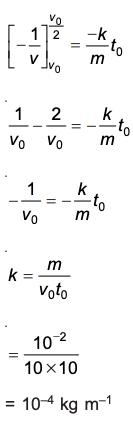Question 4: A time-dependent force F = 6t acts on a particle of mass 1 kg. If the particle starts from rest, the work done by the force during the first 1 sec. will be :

1. 1) 4.5 J
2. 2) 22 J
3. 3) 9 J
4. 4) 18 J

Solution:

6t = 1 * (dv / dt)

0v dv = ∫6t dt

v = 6 [t2 / 2]01

= 3 ms-1

W = ΔKE = (1 / 2) (1) (9) = 4.5 J

Question 5: The moment of inertia of a uniform cylinder of length l and radius R about its perpendicular bisector is I. What is the ratio l / R such that the moment of inertia is minimum?

1. 1) √(3 / 2)
2. 2) √3 / 2
3. 3) 1
4. 4) 3 / √2

Solution: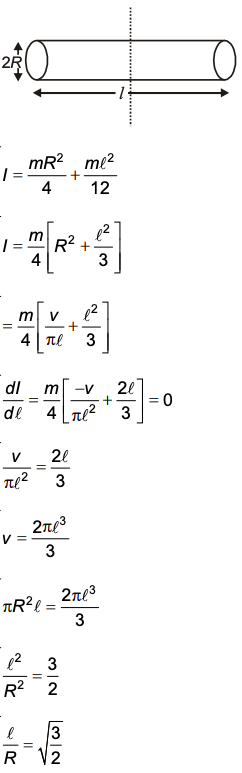Question 6: A slender uniform rod of mass M and length l is pivoted at one end so that it can rotate in a vertical plane (see figure). There is negligible friction at the pivot. The free end is held vertically above the pivot and then released. The angular acceleration of the rod when it makes an angle θ with the vertical is :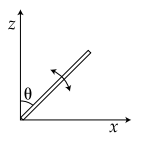1. 1) [3g / 2l] sin θ
2. 2) [2g / 3l] sin θ
3. 3) [3g / 2l] cos θ
4. 4) [2g / 3l] cos θ

Solution:

Torque at angle θ

τ = Mg sin θ (l / 2)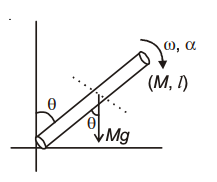τ = lɑ

lɑ = Mg sin θ (l / 2)

(Ml2 / 3) ɑ = Mg sin θ (l / 2)

(lɑ / 3) = g ((sin θ) / 2)

ɑ = [3g sin θ] / 2l

Question 7: The variation of acceleration due to gravity g with distance d from the centre of the earth is best represented by (R = Earth’s radius) :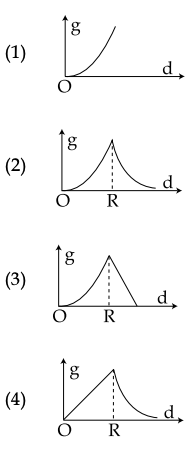Solution: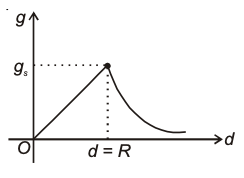Variation of g inside earth surface

d < R = g = [Gm / R2] * d

d = R = gs = Gm / R2

d > R = g = Gm / d2

Question 8: A copper ball of mass 100 gm is at a temperature T. It is dropped in a copper calorimeter of mass 100 gm, filled with 170 gm of water at room temperature. Subsequently, the temperature of the system is found to be 750C. T is given by : (Given : room temperature = 300C, specific heat of copper = 0.1 cal / gmoC)

1. 1) 800oC
2. 2) 885oC
3. 3) 1250oC
4. 4) 825oC

Solution:

100 × 0.1 × (t – 75) = 100 × 0.1 × 45 + 170 × 1 × 45

10t – 750 = 450 + 7650

10t = 1200 + 7650

10t = 8850

t = 885°C

Question 9: An external pressure P is applied on a cube at 0°C so that it is equally compressed from all sides. K is the bulk modulus of the material of the cube and ɑ is its coefficient of linear expansion. Suppose we want to bring the cube to its original size by heating. The temperature should be raised by :

1. 1) P / 3ɑK
2. 2) P / ɑK
3. 3) 3ɑ / PK
4. 4) 3PKɑ

Solution:

K = ΔP / (-ΔV / V)

ΔV / V = P / K

V = Vo (1 + γ Δt)

ΔV / Vo = γ Δt

P / K = γ Δt

Δt = P / γK = P / 3ɑK

Question 10: Cp and Cv are specific heats at constant pressure and constant volume respectively. It is observed that

Cp − Cv = a for hydrogen gas

Cp − Cv = b for nitrogen gas

The correct relation between a and b is :

1. 1) a = 1 / 14 b
2. 2) a = b
3. 3) a = 14 b
4. 4) a = 28 b

Solution:

Let molar heat capacity at constant pressure = Xp and molar heat capacity at constant volume = Xv

Xp – Xv = R

MCp – MCv = R

Cp − Cv = R / M

For hydrogen; a = R / 2

For N2; b = R / 28

a / b = 14

a = 14b

Question 11: The temperature of an open room of volume 30 m3 increases from 17oC to 27oC due to the sunshine. The atmospheric pressure in the room remains 1 × 105 Pa. If ni and nf are the number of molecules in the room before and after heating, then nf − ni will be :

1. 1) −1.61 × 1023
2. 2) 1.38 × 1023
3. 3) 2.5 × 1025
4. 4) −2.5 × 1025

Solution:

n1 = initial number of moles

n1 = P1V1 / RT1 = (105 * 30) / (8.3 * 290) = 1.24 * 103

n2 = final number of moles

n2 = P2V2 / RT2 = (105 * 30) / (8.3 * 300) = 1.20 * 103

Change of number of molecules :

nf - ni = (n2 - n1) * 6.023 * 1023

= -2.5 × 1025

Question 12: A particle is executing simple harmonic motion with a time period T. At time t = 0, it is at its position of equilibrium. The kinetic energy-time graph of the particle will look like :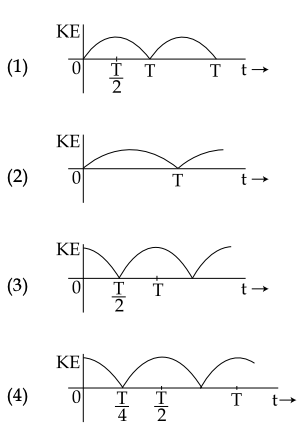Solution:

KE = (1 / 2) mω2A2cos2 ωt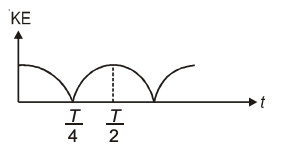Question 13: An observer is moving with half the speed of light towards a stationary microwave source emitting waves at frequency 10 GHz. What is the frequency of the microwave measured by the observer? (speed of light = 3 × 108 ms−1)

1. 1) 10.1 GHz
2. 2) 12.1 GHz
3. 3) 17.3 GHz
4. 4) 15.3 GHz

Solution:

For relativistic motion

f = fo √(c + v) / (c - v) [v = relative speed of approach]

f = 10 √(c + [c / 2]) / (c - [c / 2]) = 10√3 = 17.3 GHz

Question 14: An electric dipole has a fixed dipole moment p, which makes angle θ with respect to the x-axis. When subjected to an electric field E1 = Ei, it experiences a torque T1 = τ k. When subjected to another electric field E2 = √3 E1 j it experiences a torque T2 = - T1. The angle θ is :

1. 1) 30o
2. 2) 45o
3. 3) 60o
4. 4) 90o

Solution: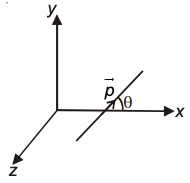p = p cos θ i + p sin θ j

E1 = Ei

T1 = p x E1 = (p cos θ i + p sin θ j) x E (i)

τ k = pE sin θ (- k) ---- (i)

E2 = √3E1 j

T2 = (p cos θ i + p sin θ j) x √3E1 j

- τ k = √3p E1cos θ k ----- (ii)

From (i) and (ii)

pE sin θ = √3pE cos θ

tan θ = √3

θ = 60o

Question 15: A capacitance of 2 µF is required in an electrical circuit across a potential difference of 1.0 kV. A large number of 1 µF capacitors are available which can withstand a potential difference of not more than 300 V. The minimum number of capacitors required to achieve this is :

1. 1) 2
2. 2) 16
3. 3) 24
4. 4) 32

Solution:

The following arrangement will do the needful: 8 capacitors of 1µF in parallel with four such branches in series.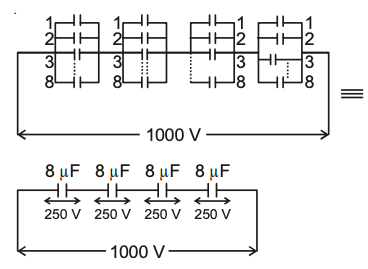Question 16: In the given circuit diagram when the current reaches steady state in the circuit, the charge on the capacitor of capacitance C will be :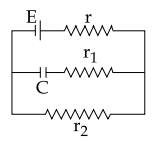1. 1) CE
2. 2) CE (r1 / r2 + r)
3. 3) CE (r2 / r + r2)
4. 4) CE (r1 / r1 + r)

Solution:

In steady-state, the flow of current through the capacitor will be zero.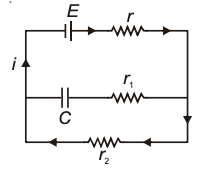i = E / (r + r2)

Vc = ir2C = Er2C / (r + r2)

Vc = CE (r2 / (r + r2))

Question 17: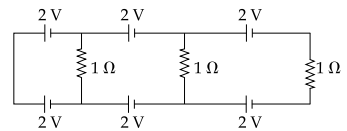In the above circuit the current in each resistance is :

1. 1) 1 A
2. 2) 0.25 A
3. 3) 0.5 A
4. 4) 0 A

Solution:

The potential difference in each loop is zero.

∴ No current will flow.

Question 18: A magnetic needle of magnetic moment 6.7 × 10−2 Am2 and moment of inertia 7.5 × 10−6 kg m2 is performing simple harmonic oscillations in a magnetic field of 0.01 T. Time taken for 10 complete oscillations is :

1. 1) 6.65 s
2. 2) 8.89 s
3. 3) 6.98 s
4. 4) 8.76 s

Solution:

T = 2π √I / MB

=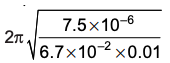= [2π / 10] * 1.06

For 10 oscillations,

t = 10T = 2π × 1.06

= 6.6568 ≈ 6.65 s

Question 19: When a current of 5 mA is passed through a galvanometer having a coil of resistance 15 Ω, it shows full-scale deflection. The value of the resistance to be put in series with the galvanometer to convert it into a voltmeter of range 0 − 10 V is :

1. 1) 1.985 × 103
2. 2) 2.045 × 103
3. 3) 2.535 × 103
4. 4) 4.005 × 103

Solution:

ig = 5 × 10–3 A

G = 15 Ω

Let series resistance be R.

V = ig (R + G)

10 = 5 × 10–3 (R + 15)

R = 2000 – 15 = 1985 = 1.985 × 103

Question 20: In a coil of resistance 100 Ω, a current is induced by changing the magnetic flux through it as shown in the figure. The magnitude of change in flux through the coil is :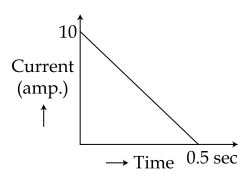1. 1) 200 Wb
2. 2) 225 Wb
3. 3) 250 Wb
4. 4) 275 Wb

Solution:

ϵ = dɸ / dt

iR = dɸ / dt

∫dɸ = R ∫i dt

Magnitude of change in flux = R × area under current vs time graph

= 100 × (1 / 2) × (1 / 2) × 10

= 250 Wb

Question 21: An electron beam is accelerated by a potential difference V to hit a metallic target to produce X-rays. It produces continuous as well as characteristic X-rays. If λmin is the smallest possible wavelength of X-ray in the spectrum, the variation of log λmin with log V is correctly represented in :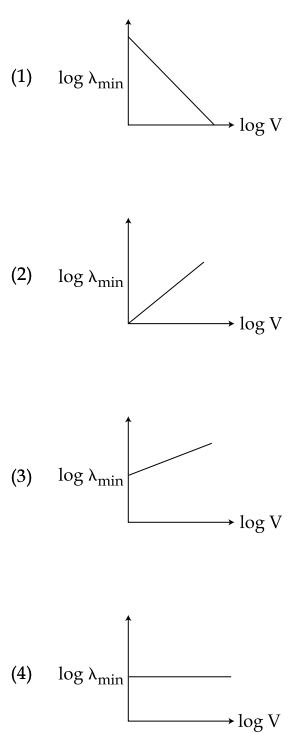Solution:

In X-ray tube

λmin = hc / eV

In λmin = ln (hc / e) - ln V

Slope is negative

Intercept on the y-axis is positive.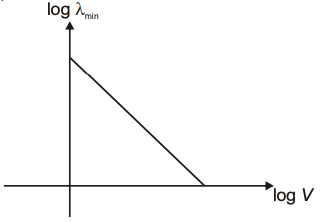Question 22: A diverging lens with a magnitude of focal length 25 cm is placed at a distance of 15 cm from a converging lens of the magnitude of focal length 20 cm. A beam of parallel light falls on the diverging lens. The final image formed is :

1. 1) real and at a distance of 40 cm from the convergent lens.
2. 2) virtual and at a distance of 40 cm from the convergent lens.
3. 3) real and at a distance of 40 cm from the divergent lens.
4. 4) real and at a distance of 6 cm from the convergent lens.

Solution: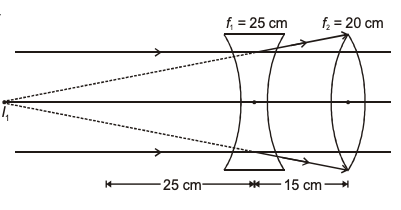For converging lens u = –40 cm which is equal to 2f

The image will be real and at a distance of 40 cm from a convergent lens.

Question 23: In Young’s double-slit experiment, slits are separated by 0.5 mm, and the screen is placed 150 cm away. A beam of light consisting of two wavelengths, 650 nm and 520 nm, is used to obtain interference fringes on the screen. The least distance from the common central maximum to the point where the bright fringes due to both the wavelengths coincide is :

1. 1) 1.56 mm
2. 2) 7.8 mm
3. 3) 9.75 mm
4. 4) 15.6 mm

Solution:

For λ1, y = mλ1D / d

For λ2, y = nλ2D / d

m / n = λ2 / λ1 = 4 / 5

For λ1,

y = mλ1D / d

λ1 = 650 nm = 7.8 nm

Question 24: A particle A of mass m and initial velocity v collides with a particle B of mass m / 2 which is at rest. The collision is head on, and elastic. The ratio of the de-Broglie wavelengths λA to λB after the collision is :

1. 1) λA / λB = 1/3
2. 2) λA / λB = 2
3. 3) λA / λB = 2/3
4. 4) λA / λB = 1/2

Solution:

v1 = [(m1 - m2)v / (m1 + m2)] + 0 = v / 3

p1 = m [v / 3]

v2 = [2m1v / (m1 + m2)] + 0 = 4v / 3

p2 = (m / 2) (4v / 3) = 2mv / 3

Therefore, de-Broglie wavelength (λA / λB) = p2 / p1 = 2:1

Question 25: Some energy levels of a molecule are shown in the figure. The ratio of the wavelengths r = λ1 / λ2, is given by :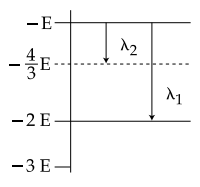1. 1) r = 4/3
2. 2) r = 2/3
3. 3) r = 3/4
4. 4) r = 1/3

Solution:

From energy level diagram

λ1 = hc/E

λ2 = hc/(E/3)

λ1 / λ2 = 1/ 3

Question 26: A radioactive nucleus A with a half life T, decays into a nucleus B. At t = 0, there is no nucleus B. At some time t, the ratio of the number of B to that of A is 0.3. Then, t is given by :

1. 1) t = (T / 2) (log 2 / log 1.3)
2. 2) t = (T) (log 1.3 / log 2)
3. 3) t = T log (1.3)
4. 4) t = T / [log (1.3)]

Solution:

[No - Noe-λt] / [Noe-λt] = 0.3

eλt = 1.3

λt = ln 1.3

(ln 2 / T) t = ln 1.3

t = T * (ln (1.3)) / ln 2

t = T * (log (1.3)) / log 2

Question 27: In a common emitter amplifier circuit using an n-p-n transistor, the phase difference between the input and the output voltages will be :

1. 1) 45o
2. 2) 90o
3. 3) 135o
4. 4) 180o

Solution:

In common emitter configuration for the n-p-n transistor, the phase difference between output and input voltage is 180°.

Question 28: In amplitude modulation, sinusoidal carrier frequency used is denoted by ωc and the signal frequency is denoted by ωm. The bandwidth (∆ωm) of the signal is such that ∆ωm << ωc. Which of the following frequencies is not contained in the modulated wave?

1. 1) ωm
2. 2) ωc
3. 3) ωm + ωc
4. 4) ωc − ωm

Solution:

Modulated wave has a frequency range.

ωc ± ωm

Since, ωc >> ωm

Hence, ωm is excluded.

Question 29: Which of the following statements is false?

1. 1) Wheatstone bridge is the most sensitive when all the four resistances are of the same order of magnitude.
2. 2) In a balanced Wheatstone bridge if the cell and the galvanometer are exchanged, the null point is disturbed.
3. 3) A rheostat can be used as a potential divider.
4. 4) Kirchhoff’s second law represents energy conservation.

Solution:

In a balanced Wheatstone bridge, the null point remains unchanged even if the cell and galvanometer are interchanged.

Question 30: The following observations were taken for determining surface tension T of water by capillary method : diameter of capillary, D = 1.25 × 10−2 m rise of water, h = 1.45 × 10−2 m. Using g = 9.80 m/s2 and the simplified relation T = [rhg / 2] × 103 N / m, the possible error in surface tension is closest to :

1. 1) 0.15%
2. 2) 1.5%
3. 3) 2.4%
4. 4) 10%

Solution:

[ΔT / T] * 100 = [ΔD / D] * 100 + [Δh / h] * 100

= (0.01 / 1.25) * 100 = (0.01 / 1.45) * 100

= (100 / 125) + (100 / 145)

= 0.8 + 0.689

= 1.489

= 1.5%

### JEE Main 2017 Physics Paper With Solutions 2nd April